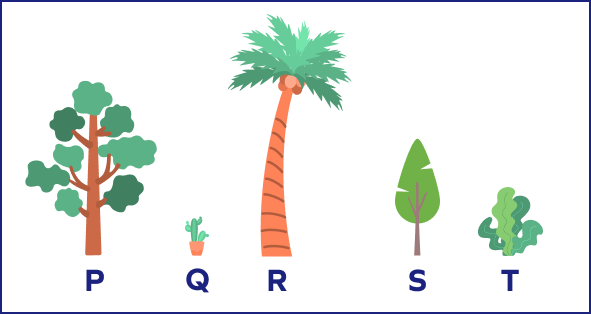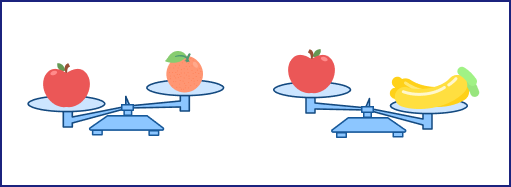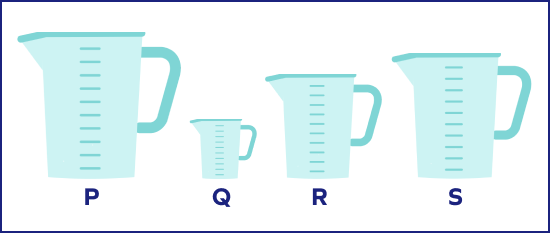# Sorting in Math – Definition, Examples, Facts

## What is Sorting in Math? Introduction

Have you ever tried organizing your legos? How do you do that? You “sort” the parts either by color or shape! So, what is the concept of ‘sorting’ in math?

Sorting is the process where we are able to identify the similarities or the differences among a set of objects to arrange them in a defined sequence.

## Sorting: Definition

Sorting can be defined as the process of ordering, comparing, contrasting and systematically grouping or organizing different items. Sorting can be done easily on the basis of color, size, texture, etc.

There are many math sorting activities that also help students with daily tasks, like separating silverware, packing groceries, or doing laundry. The following image shows an example of sorting marbles based on their colors.

## Different Ways of Sorting

We can sort the objects or the shapes based on color, shape, size, texture, etc.

### Sorting by Size

Objects or shapes can be sorted according to how big or small they are.

The circles given below are sorted according to their size, from largest to smallest.

### Sort by Height

To sort the objects from shortest to longest, we follow these steps:

Step 1: Look through all the items and see which of them is the shortest of all. This item will go first on your sorted list.

Step 2: Look through the remaining items to find the shortest after that. Add it to your sorted list.

Step 3: Repeat step 2 until all items are sorted.

Let us look at some pencils and arrange them from shortest to longest.

The pencils sorted from the shortest to longest the order is: Pencil P, Pencil R, Pencil Q, Pencil S.

We can also use the comparison symbols which are $\lt$ and $\gt$ to show the sorted list of pencils.

We can say Pencil P $\lt$ Pencil R $\lt$ Pencil Q $\lt$ Pencil S.

Algorithms are like computer programs. They are a set of steps that are used to solve a problem. Such a sorting algorithm is known as “Bubble sort.”

An object is said to be the tallest if it has the biggest height in comparison to other objects and an object is said to be the shortest if it has the smallest height in comparison to other objects.

An example of sorting by height (shortest to tallest):

One more example of sorting by height (tallest to shortest):

### Sort by Colors

When we sort the objects by colors, we keep the shapes or objects of the same color in one group.

In the image given below, the shapes are sorted by colors. The green shapes are together, yellow shapes in another bunch, orange shapes in the third and purple shapes in the fourth.

Look at the figure given below; all rings of the same color are placed together.

### Sort by Shapes

When we sort by shape, we keep the same shapes together irrespective of their size or color. In the image given below, there are different shapes.

We see there are four different shapes based on the properties: triangle, rectangle, square, and circle. So, we place all triangles together, all rectangles together, all squares together and all circles together.

On sorting according to the shapes, we get:

## Sorting vs. Matching

Matching is the process of identifying two similar things based on their shared characteristics. We can match objects based on their color, size, form, quantity, etc.

Sorting is the process of finding similarities and differences among a collection of objects.

Pairing two objects based on a particular common characteristic is called matching. Grouping a number of objects together is called sorting.

## Ascending and Descending Order

We can sort the numbers in ascending or descending order. Ascending order means to sort the numbers from smallest to greatest. Descending order means to sort the numbers from greatest to smallest.

Let us take an example of four friends: Sophie with 157 cm height, Charlie with 162 cm height, Jacob with 143 cm and Mark with 139 cm height.

On sorting the heights in ascending order, we get: $139$ cm $\lt 143$ cm $\lt 157$ cm $\lt 162$ cm

On sorting the heights in descending order, we get $162$ cm $\gt 157$ cm $\gt 143$ cm $\gt 139$ cm.

## Sorting Numbers by Number of Digits

We can also sort the numbers on the basis of the number of digits.

Single-digit numbers are $0 ​​− 9$.

Double-digit Numbers are $10 − 99$.

Three-digit Numbers are $100 − 999$.

## Conclusion

In this article, we learned about sorting. Sorting means identifying the similarities and differences between two or more objects and shapes. Let’s solve some examples for better understanding.

## Solved Examples on Sorting

1. Count and sort the fruits in separate groups.

Solution: On counting, we get there are 24 fruits.

Number of apples $= 5$

Number of guava $= 9$

Number of avocados $= 7$

Number of mangoes $= 3$

2. Sort the numbers on the basis of the number of digits.

24, 105, 146, 75, 92, 113, 178, 195, 46, 50

How many three-digit numbers are there?

Solution:

The two-digit numbers are 24, 75, 92, 46, 50

The three-digit numbers are 105, 146, 113, 178, 195

Number of digit numbers $= 5$

3. Sort the objects on the basis of color.

Solution:

Objects of red color $=$ Cherries, Crab

Objects of yellow color $=$ Banana, Yellow Capsicum

Objects of green color $=$ Whale, Butterfly

4. Michael is 23 years old, Ella is 16 years old, George is 19 years old, and Sam is 21 years old. Sort them from youngest to oldest.

Solution:

Firstly, we will sort the numbers in ascending order.

16 years $\lt$ 19 years $\lt$ 21 years $\lt$ 23 years

So, from youngest to oldest, we get: Ella, George, Sam, Michael.

5. Sort the objects from thinnest to thickest.

Solution:

The thinnest book has the minimum thickness, i.e., of book R.

The book with thickness more than Book R is Book P.

The thickest book is Book P.

So, from thinnest to thickest, we get Book R, Book P, Book Q.

## Practice Problems

1

### Numbers: 11, 22, 43, 106, 87, 97, 64, 52, 103 How many even numbers are there?

1
2
5
4
CorrectIncorrect
Even numbers are the numbers that are divisible by 2.
Even Numbers: 22, 106, 64, 52
There are 4 even numbers.
2

### When sorting the following plants/trees on the basis of tallest to shortest, which of the following will come in the middle?Tree P
Tree S
Plant Q
Plant T
CorrectIncorrect
The tallest tree is R. Plant Q is the shortest.
Tree P is shorter than Tree R but taller than the Q, S and T.
Tree S is shorter than Tree P but taller than T and Q.
Plant T is shorter than Tree S but taller than plant Q.
On sorting from tallest to shortest, we get: Tree R $\gt$ Tree P $\gt$ Tree S $\gt$ Plant T $\gt$ Plant Q
3

### Sort the objects from lightest to heaviest.Orange, Apple, Bananas
Orange, Bananas, Apple
Apple, Bananas, Orange
Apple, Orange, Bananas
CorrectIncorrect
Correct answer is: Orange, Apple, Bananas
The first figure says that the orange is lighter than the apple.
The second figure says that the apple is lighter than bananas.
So, from lightest to heaviest the order is: Orange, Apple, Bananas.
4

### On sorting the jugs based on low capacity to high capacity, which beaker has the least capacity?Jug P
Jug R
Jug Q
Jug S
CorrectIncorrect